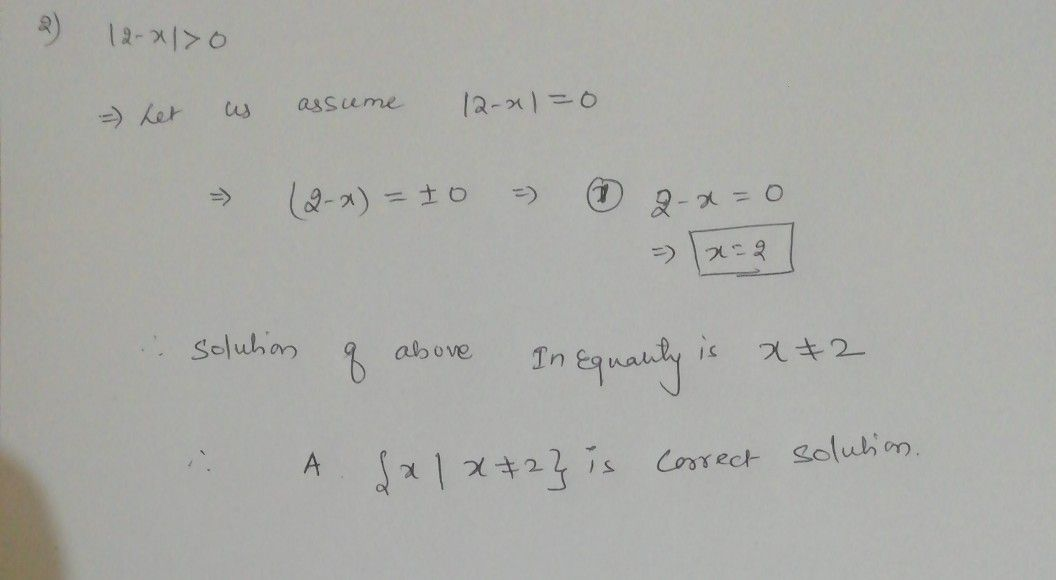Symbol
Problem$2.$ Himpunan penyelesaian pertidaksamaan $|2-x>0$ adalah. . . . A. $\left(x|x≠2\right)$ B. $4x1x>6\right)$ C. $\left(x1-2<x<6\right)$ D. $\left(x1x<-2$ atau $x>4\right)$ E. $\left(x1x<-2$ atau $x>6\right)$
Other
Search count: 106
SolutionQanda teacher - anku1911For any value of x except 2, above inequality will be true
If you need any further assistance regarding the above solution, please feel free to contact me
If you are satisfied with the answer, kindly evaluate and rate the answer. Thank you very muchStudent
wow thank you for helping meQanda teacher - anku1911
You are welcome